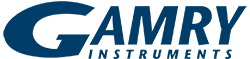# Determination of the correct value of Cdl from the impedance results fitted by the commercially available software

#### IntroductionWith the introduction of a constant phase element (CPE) as a replacement for the capacity in EIS measurements, most of the experimentally obtained results could be fitted by commercially available software (Gamry, Scribner, Solartron, etc.). Much better fit results were obtained with CPE in comparison with the fit results obtained by using pure capacity, represented by parallel plate condenser. Taking into account that the CPE defines inhomogeneity of the surface in the electrochemical EIS experiments and inhomogeneity of the charge distribution in solid state EIS measurements, it is reasonable to expect that better fit for real systems is obtained by using CPE. The main problem in the use of commercially available software with CPE is the fact that the capacity, obtained by fitting procedure, does not have the dimension of capacity, i.e., F cm-2, or Ω-1cm-2 s, but its dimension is given in Ω-1 cm-2 sa, where a is the exponent in the equation for the CPE  ( ZCPE = Zdl(jω)-a ).

This problem has been discussed in few papers [2-4] and recently Hsu and Mansfeld  developed the equation for correction of capacity to its real value, in the case of parallel connection of CPE and R, by using equation (1).where ω”max represents the frequency of the maximum on the -Z’’ vs. ω dependence, which is independent of the exponent a, while Adl represents the result of fitting.

While discussing different procedures for the treatment of the distribution of time constants, M. Orazem, et. al.  pointed out that the impedance of an equivalent circuit for parallel connection of CPE and R can be expressed by either equation (2) or equation (3)It is important to note that equation (3) is used in all commercially available software for fitting impedance spectra.

Most recently it was shown for Ag(111) in 0.01 M NaCl  and Cu single crystals in 0.1 M NaOH  that even single crystal surfaces cannot be treated as being homogeneous and that, instead of Cdl, CPE should be introduced in the analysis of Cdiff versus ƒ curves for the adsorption of investigated anions. Equation (4) is obtained  by replacing Cdl by a CPE, and using the commercial software definition of a CPE.Since in this equation Adl does not have the dimensions of capacity, it was necessary to correct the value of Adl obtained by fitting experimental Cdiff versus ƒ curves. According to G. J. Brug, et. al. , for a series connection of the CPE and Rs (solution resistance), the value of Cdl should be defined by equation (5) .In this work the analysis of the differential capacity and the impedance of the electrochemical process corresponding to the parallel connection of CPE and R is performed in order to define the relation between the real value of the capacity and the value obtained by fitting EIS results using commercially available software.

#### Results and discussion

Considering equations (2) and (3), it can be concluded that the former one, equation (2), seems to be more correct, since in this equation the frequency dispersion of the capacity, as a consequence of the surface inhomogeneity, is closely related to the charge transfer distribution over the same surface and these two factors are mutually dependent. The differential capacity derived from equation (2) is:The differential capacity derived from equation (3) is:Hence, equation (6) is dimensionally correct, while equation (7) is dimensionally incorrect, i.e., additional correction is necessary.

Simultaneously the real (Z’) and the imaginary (Z’’) component of the impedance are defined by equations (8) and (9) respectively.In the case that CPE is considered as independent parameter, as in commercially available software (equation (3)), equation for the differential capacity is given by equation (7), while Z’ and Z’’ are defined by equations (10) and (11) respectively and are dimensionally incorrect.Using equations (8) and (9) it can be shown that Z’-Z’’ diagrams (Fig. 1) are identical to the ones obtained by using equations (10) and (11), but there is significant difference in the shape of -Z’’ vs. log f for these two cases. As it is mentioned in the paper of Hsu and Mansfeld , when impedance equations (8) and (9) are used the maximum on the -Z’’ vs. log ƒ is independent of frequency (Fig. 2). After fitting experimental results by commercially available software, the correct value of the double layer capacity can be obtained by using equation (1).The difference between the correct and fitted value of the capacity could be significant and this difference depends on the values of R and a, as shown in Fig. 3.

#### Conclusion

From the results and discussion it could be concluded that only in the case of parallel connection of CPE and R correction for the real value of the double layer capacity by using equation (1) is valid.#### References

1. J. R. Macdonald, Impedance Spectroscopy Emphasizing Solid Materials and Systems, Wiley, New York, Chichester, Brisbane, Toronto, Singapore, 1987.
2. E. van Westing, PhD Thesis, Technical University of Delft, 1992.
3. S.F. Mertens, C. Xhoffer, B.C. De Cooman and E. Temmerman, Corrosion 53 (1997) 381.
4. G.O. Ilevbare, J.R. Scully, Corrosion 39 (1983) 466.
5. C.H. Hsu and F. Mansfeld, Corrosion 57 (2001) 747.
6. M.E. Orazem, P. Shukla and M.A. Membrino, Electrochim. Acta 47 (2002) 2027.
7. V. D. Jovic and B.M. Jovic, J. Electroanal. Chem. 541 (2003) 1.
8. V. D. Jovic and B.M. Jovic, J. Electroanal. Chem. 541 (2003) 12.
9. G.J. Brug, A.L.G. van Eeden, M. Sluyters-Rehbach and J. Sluyters, J. Electroanal. Chem. 176 (1984) 275.

V. D. Jovic
Center for Multidisciplinary Studies,